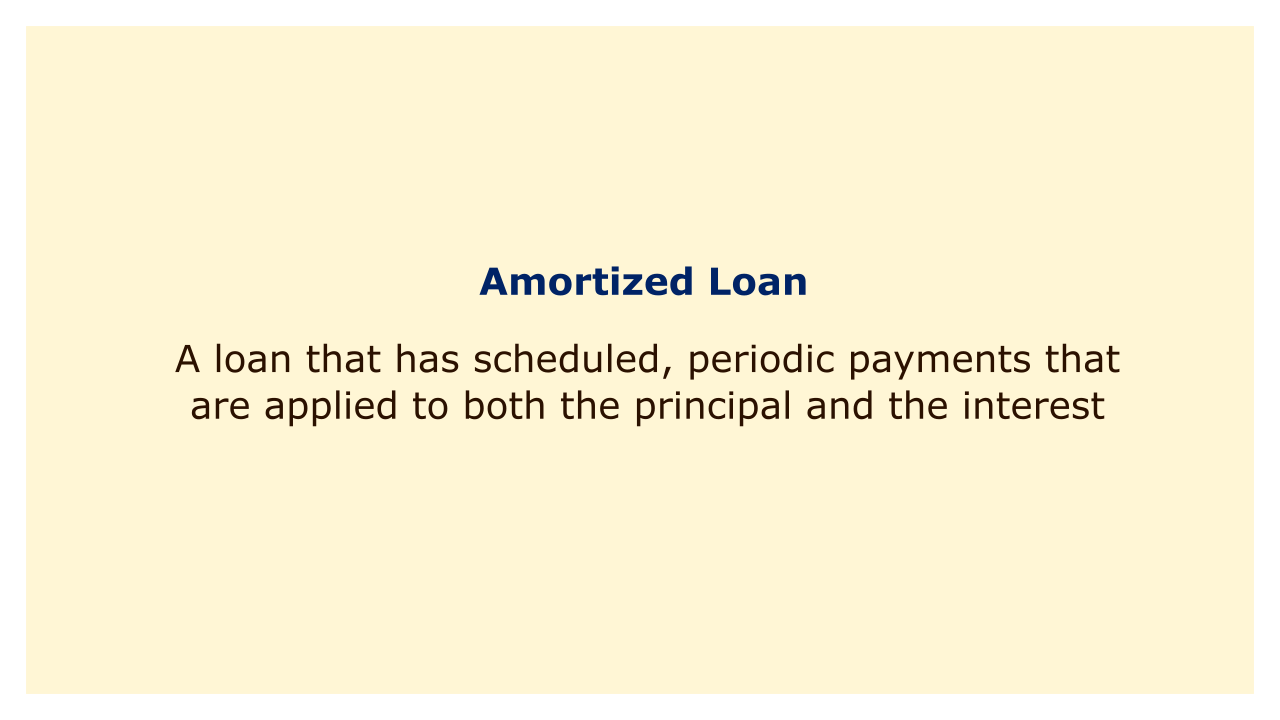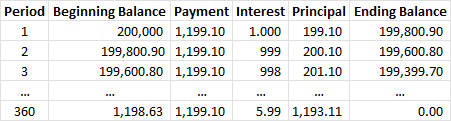# Amortized LoanImage: Moneybestpal.com

### The term "amortized loan" refers to a loan with predetermined, regular payments that are applied to both the principal and the interest. The amount of each payment depends on the loan amount, the interest rate, and the loan duration.

#### How Amortized Loans Work

An amortized loan is one that has its principal and interest paid off over a certain length of time, typically monthly. The loan's present value, which is determined by the interest rate and the number of payments, is divided by the annuity factor to determine the payment amount. The formula for the payment is:

Payment = Present Value / Annuity Factor

The present value is the amount of money borrowed or owed at the beginning of the loan. The annuity factor is a factor that converts a series of equal payments into a present value. The annuity factor can be found using a financial calculator or a table, or by using this formula:

Annuity Factor = (1 - 1 / (1 + i)^n) / i

where i is the periodic interest rate (annual interest rate divided by number of payments per year) and n is the total number of payments.

For example, suppose you borrow \$200,000 to buy a house at a 6% annual interest rate for 30 years. The monthly payment can be calculated as follows:

Present Value = \$200,000

Annuity Factor = (1 - 1 / (1 + 0.06 / 12)^360) / (0.06 / 12) = 166.7916

Payment = \$200,000 / 166.7916 = \$1,199.10

This means that you have to pay \$1,199.10 every month for 30 years to pay off the loan.

#### How to Calculate the Amortization Schedule

An amortization schedule is a table that displays the principle and interest components of each payment as well as the evolution of the loan total over time. You need to know the loan balance, interest rate, and payment amount for each period in order to compute the amortization plan.

The loan sum at the start of each period is multiplied by the periodic interest rate to determine the interest for that period. The interest is deducted from the payment to determine the principle for each period. The principle is deducted from the loan balance at the start of each period to determine the loan balance at the conclusion of that period.

For example, using the same loan as above, the first few rows of the amortization schedule would look like this:Image: Moneybestpal.com

The table shows that each payment's interest component lowers with time while its principal component grows. This implies that when you pay off your loan, a larger portion of your payment will go toward decreasing your debt.

#### Examples of Amortized Loans

Amortized loans are very common in personal finance and business finance. Some examples of amortized loans are:
• Mortgage loans: These are loans used to buy real estate property, such as a house or a condo. Mortgage loans usually have long terms (15 to 30 years) and fixed or variable interest rates.
• Auto loans: These are loans used to buy vehicles, such as cars or trucks. Auto loans usually have shorter terms (3 to 7 years) and fixed interest rates.
• Personal loans: These are loans used for various purposes, such as debt consolidation, home improvement, or medical expenses. Personal loans usually have medium terms (1 to 5 years) and fixed or variable interest rates.

Amortized loans might assist you in financing significant purchases or investments over time while making regular and predictable monthly payments. Nevertheless, amortized loans also have certain disadvantages, such as paying more interest overall and having fewer options for modifying the period or amount of your payments. Consequently, you should weigh your alternatives carefully and select the one that best meets your interests and financial situation before taking out an amortized loan.
Tags